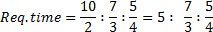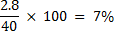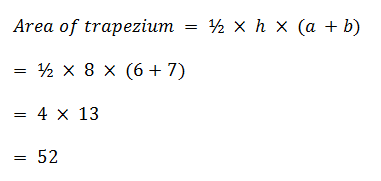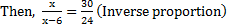## Ratio and Proportion For SBI PO : Set – 01

1) On teachers’ day sweets were to be equally distributed amongst 400 children. But on that particular day 50 children remained absent; hence each child got 1 sweet extra. How many sweets was each child originally supposed to get?

a) 8

b) 10

c) 9

d) 7

e) Cannot be determined

(d)

Let originally each child get x sweet

X×400=(x+1) 350

X=7

2) Three persons A, B and C invested capitals in the ratio 2 : 3 : 4. At the end of the business term, they received the profits in the ratio 10 : 7 : 5. What is the ratio of time for which they contributed their capital?

a) 12 : 14 : 15

b) 12 : 10 : 9

c) 5:4:1

d) 4 : 3 : 1

e) None of these

e)Ratio = 60 : 28 : 15

3) One year ago the ratio between Laxman and Gopal salary was 3:4. The individual ratios between their last year’s and this year’s salary are 4:5 and 2:3 respectively. At present the total of their salary is Rs.4160. The salary of laxman now is?

a) 1800

b) 1500

c) 2160

d) 2560

e) 1600

(e

The ratio between salary  one year ago was Laxman: Gopal=3:4

Comparing last year and current year salary of laxman,

4->3

5->(3×5)/6

Comparing last year and current year salary of Gopal,

2->4

3->(4×3)/2

Thus the ratio becomes, 5/4:2

5:8

13 parts of salary is 4160

5 parts of salary is 1600.

4) Manoj expenditure and savings are in the ratio 3 : 2. His income increases by 10%. His expenditure also increases by 12%. By how much per cent do her savings increase ?

a) 7%

b) 9%

c) 10%

d) 13%

e) None of these

(a)

Let expenditure be Rs 60 and savings be Rs 40.

Total income = Rs 100

New income = Rs 110

New expenditure = Rs 67.2

New saving = 110 – 67.2 = Rs 42.2

Percentage increase in saving5) Two parallel sides of a trapezium are 6 cm and 7 cm respectively. The perpendicular distance between the parallel sides is 8 cm. Find the area of the trapezium.?

a) 50 cm2

b) 52 cm2

c) 54 cm2

d) 56 cm2

e) 58 cm2

(b)6) If a carton containing a dozen mirrors is dropped, which of the following cannot be the ratio of the broken mirror to the unbroken mirror?

a) 2 :1

b) 7:5

c) 3:2

d) 1:5

e) None of these

c)

The carton contains a dozen mirror Hence, when dropped, a few mirrors may break. Here, the ratio obtained, no matter whatever is the number of broken mirrors, will always 8um up its terms such that they divide 12 exactly. From the given choices, we add up terms of each ratio to check, if they divide 12 or not.

For 2 : 1,        2 + 1 = 3, which divides 12

For 7 : 5, 7 + 5 = 12, which divides 12

For 1 : 5, 1 + 5 = 6, which divides 12

For 11 : 1, 11 + 1 = 12, which divides 12

But for 3 : 2,   3 + 2 = 5, which does not divides 12.

Hence, 3 : 2 cannot be the ratio.

7) Rani enters a shop to buy icecreams, cookies and pastries. She has to buy atleast 9 units of each. She buys more cookies than ice-creams and more pastries than cookies. She picks up a total of 32 items. How many cookies does she buy ?

a) Either 12 or 13

b) Either 11 or 12

c) Either 10 or 11

d) Either 9 or 11

e) Either 9 or 10

c)

Ice-creams + Cookies + Pastries = 32

9+11+12=32

or, 9+10+13= 32

10+11+12 not equal to 32

8) A group of men undertake to complete a job in 24 days but 6 of them become absent. Rest of the group complete the work in 30 days. Find the original number of men in the group?

a) 28

b) 29

c) 30

d) 31

e) None of these

c)

Let the original number of men in the group be x24x=30-180-> x=30

9) A factory employs skilled workers, unskilled workers and clerks in the proportion 8 : 5 : 1 and the wages of a skilled worker as unskilled worker and a clerk are in the ratio 5 : 2 : 3. When 20 unskilled workers are employed, the total daily wages of all, amount to Rs. 318. Find the daily wages paid to each category of employees (Rs.) :

a) 240, 57, 19

b) 210,17,13

c) 230,65,12

d) 210,17,15

e) 240,60,18

e)

No.of employees in the ratio = 8:5:1

Their wages = 5:2:3

Their cumulative salary = 40:10:3

Daily wage of clerk = {3/53}*318=18

Daily wage of skilled worker = {40/53}*318= 240

Daily wage of unskilled worker = {10/53}*318= 60

10) The total salary of A, B and C is Rs. 906. If they spend 80%, 85%, 75% of their salaries respectively, their savings are as 7:6:19. Then salary of B is

a) Rs 140

b) Rs 160

c) Rs 144

d) Rs 225

e) Rs. 240

e)

They spend 80%, 85%, 75% of their salary

So, they save 20%,15%, 25% and savings are in the ratio 7:6:19

20% of x = 7a

x=35a

similarly 15% of y= 6a

y=40a

similarly 25% of z= 19a

z=76a

Then the salary of B = {40a/151a}*906

Rs.240